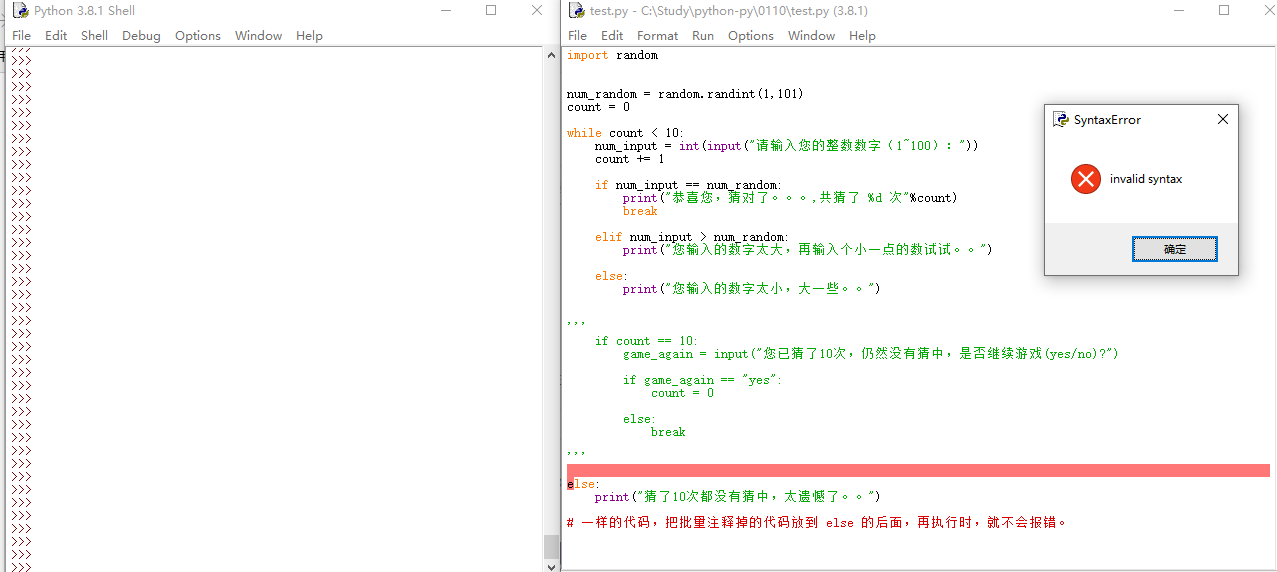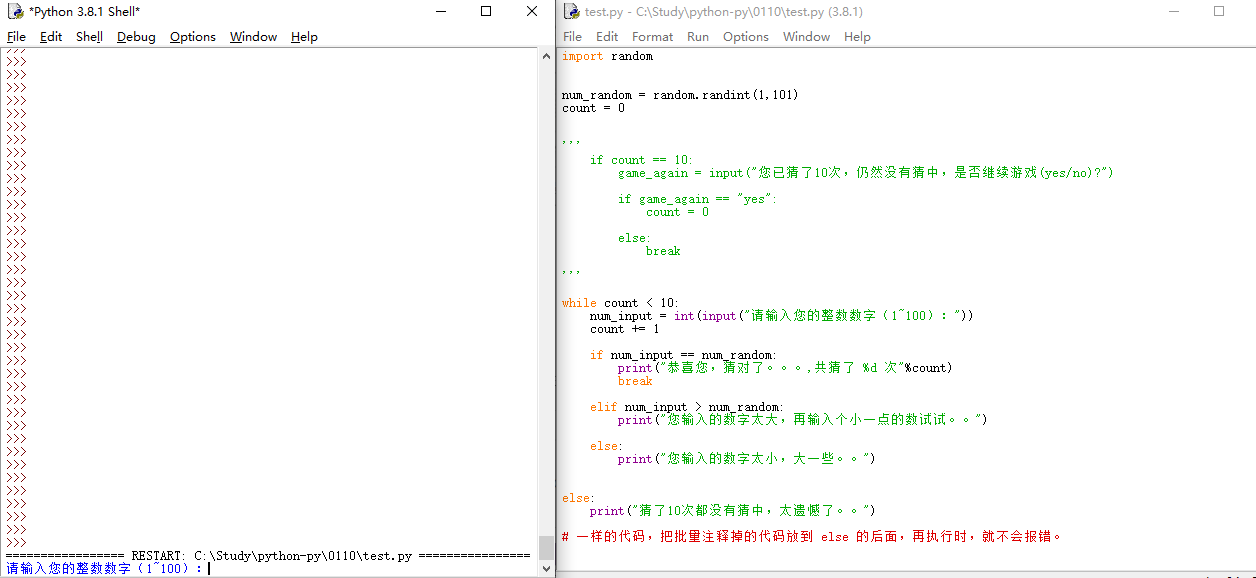•闪存
•博客
• 发言小组
• 投递新闻
• 提问博问
• 添加收藏
• 发布招聘
•文库

# python的批量注释，会导致 while... else... 中的 else 报 invalid syntax 错误，不知原因，有高手见到还请赐教

0
[已解决问题] 解决于 2020-01-10 17:19``````import random

num_random = random.randint(1,101)
count = 0

while count < 10:
num_input = int(input("请输入您的整数数字（1~100）："))
count += 1

if num_input == num_random:
print("恭喜您，猜对了。。。,共猜了 %d 次"%count)
break

elif num_input > num_random:
print("您输入的数字太大，再输入个小一点的数试试。。")

else:
print("您输入的数字太小，大一些。。")

# 使用"#"注释，执行时，下面 else 语句不会报错。
#    if count == 10:
#
#        game_again = input("您已猜了10次，仍然没有猜中，是否继续游戏(yes/no)?")
#
#        if game_again == "yes":
#            count = 0
#
#        else:
#            break

else:
print("猜了10次都没有猜中，太遗憾了。。")``````

``````import random

num_random = random.randint(1,101)
count = 0

while count < 10:
num_input = int(input("请输入您的整数数字（1~100）："))
count += 1

if num_input == num_random:
print("恭喜您，猜对了。。。,共猜了 %d 次"%count)
break

elif num_input > num_random:
print("您输入的数字太大，再输入个小一点的数试试。。")

else:
print("您输入的数字太小，大一些。。")

# 使用单引号或者双引号批量注释时，执行时，下面的 else 就会报invalid syntax 错误。

'''
if count == 10:
game_again = input("您已猜了10次，仍然没有猜中，是否继续游戏(yes/no)?")

if game_again == "yes":
count = 0

else:
break
'''

else:
print("猜了10次都没有猜中，太遗憾了。。")``````

``````import random

num_random = random.randint(1,101)
count = 0

while count < 10:
num_input = int(input("请输入您的整数数字（1~100）："))
count += 1

if num_input == num_random:
print("恭喜您，猜对了。。。,共猜了 %d 次"%count)
break

elif num_input > num_random:
print("您输入的数字太大，再输入个小一点的数试试。。")

else:
print("您输入的数字太小，大一些。。")

else:
print("猜了10次都没有猜中，太遗憾了。。")

# 一样的代码，把批量注释掉的代码放到 else 的后面，再执行时，就不会报错。

'''
if count == 10:
game_again = input("您已猜了10次，仍然没有猜中，是否继续游戏(yes/no)?")

if game_again == "yes":
count = 0

else:
break

'''``````

Python 3.8.1 (tags/v3.8.1:1b293b6, Dec 18 2019, 23:11:46) [MSC v.1916 64 bit (AMD64)] on win32

0

jakio6 | 小虾三级 |园豆：1213 | 2020-01-10 16:31
``````'''
if count == 10:
game_again = input("您已猜了10次，仍然没有猜中，是否继续游戏(yes/no)?")

if game_again == "yes":
count = 0

else:
break

'''``````@小蜻蜓点水: 字符串不是注释，就跟个没有作用的语句一样. 你个什么语句到那里都会报错啊

jakio6 | 园豆：1213 (小虾三级) | 2020-01-10 17:00

@小蜻蜓点水: 在正常可以出现语句的地方可以当做注释用，但是不能说它就和注释是等价的

jakio6 | 园豆：1213 (小虾三级) | 2020-01-10 17:02

@jakio6: 我明白了，python中单双引号是被认为是字符串的，可以用作注释用，这次主要原因是因为 while 代码块 和 else 代码块 之间不能有其他代码了（纯注释代码除外），所以才报错。

您需要登录以后才能回答，未注册用户请先注册